2018-12-09 19:41:37 freehawkzk 阅读数 882
• ###### 数据结构基础系列(2)：线性表

数据结构课程是计算机类专业的专业基础课程，在IT人才培养中，起着重要的作用。课程按照大学计算机类专业课程大纲的要求，安排教学内容，满足需要系统学习数据结构的人。系列课程包含11个部分，本课为第2部分，线性表的逻辑结构，线性表的顺序表和链表两种存储结构，以及在各种存储结构中基本运算的实现，还通过相关的应用案例介绍了相关知识的应用方法。

19186 人正在学习 去看看 贺利坚

# 1 概念

灰度线性变换是一种灰度变换，通过建立灰度映射来调整源图像的灰度，达到图像增强的目的。灰度映射通常使用灰度变换曲线来表示。

# 2 原理

灰度线性变换就是将图像的像素值通过指定的线性函数进行变换，以此增强或减弱图像的灰度。灰度线性变换的公式是常见的一维线性函数：
$g(x,y) = k \cdot f(x,y) + b$
$x$为原始灰度值，则变换后的灰度值$y$为：
$y = k \cdot x + b \dots\dots(0 \leq y \leq 255)$
$k$表示直线的斜率，即倾斜程度，$b$表示线性函数在$y$轴的截距。

# 3 作用

$k$ $b$取值 意义
$k>1$ 增大图像的对比度，图像的像素值在变换后全部增大，整体效果被增强
$k=1$ 通过调整$b$，实现对图像亮度的调整
$0 < k < 1$ 图像的对比度被削弱
$k<0$ 原来图像亮的区域变暗，原来图像暗的区域变亮

# 4 Matlab实现

clc;
clear;
close all;

% 对灰度图进行灰度线性变换
ori_img = rgb2gray(ori_img);
[oriHist,oriX] = imhist(ori_img);

k = 1.25;
d = 0;
gray1 = ori_img * k + d;
[g1Hist,g1X] = imhist(gray1);

k = 1;
d = 50;
gray2 = ori_img * k + d;
[g2Hist,g2X] = imhist(gray2);

k = 0.5;
d = 0;
gray3 = ori_img * k + d;
[g3Hist,g3X] = imhist(gray3);

k = -1;
d = 255;
ori_ = im2double(ori_img);
gray4 = ori_ * k + 1.0;
[g4Hist,g4X] = imhist(gray4);

figure(1),subplot(1,2,1),imshow(ori_img),title('原图');subplot(1,2,2),imshow(gray1),title('k>0 d=0');
figure(2),subplot(1,2,1),stem(oriX,oriHist),title('原图直方图');subplot(1,2,2),stem(g1X,g1Hist),title('k>0 d=0直方图');
figure(3),subplot(1,2,1),imshow(ori_img),title('原图');subplot(1,2,2),imshow(gray2),title('k=1 d=50');
figure(4),subplot(1,2,1),stem(oriX,oriHist),title('原图直方图');subplot(1,2,2),stem(g2X,g2Hist),title('k=1 d=50直方图');
figure(5),subplot(1,2,1),imshow(ori_img),title('原图');subplot(1,2,2),imshow(gray3),title('k=0.5 d=0');
figure(6),subplot(1,2,1),stem(oriX,oriHist),title('原图直方图');subplot(1,2,2),stem(g3X,g3Hist),title('k=0.5 d=0直方图');
figure(7),subplot(1,2,1),imshow(ori_img),title('原图');subplot(1,2,2),imshow(gray4),title('k=-1 d=255');
figure(8),subplot(1,2,1),stem(oriX,oriHist),title('原图直方图');subplot(1,2,2),stem(g4X,g4Hist),title('k=-1 d=255直方图');


# 5 OpenCV实现

#include <iostream>
#include <string>

#include "../include/opencv400/opencv2/opencv.hpp"
#include "windows.h"

std::string g_CurrentDirectory;
void SetCurrentDirectoryToExePath()
{
HMODULE hExe = GetModuleHandleA(NULL);
char nameBuf[MAX_PATH] = { 0 };
GetModuleFileNameA(hExe, nameBuf, MAX_PATH);
std::string sName(nameBuf);
sName = sName.substr(0, sName.rfind('\\'));
SetCurrentDirectoryA(sName.c_str());
g_CurrentDirectory = sName;
}

void calcHist1D(cv::Mat& input, cv::Mat& output)
{
int channels[] = { 0 };
int histsize[] = { 256 };
float grayRnage[] = { 0,256 };
const float* ranges[] = { grayRnage };
cv::MatND hist;
cv::calcHist(&input, 1, channels, cv::Mat(), hist, 1, histsize, ranges);

double maxVal = 0;
cv::minMaxLoc(hist, 0, &maxVal, 0, 0);

int scale = 10;
output = cv::Mat::zeros(500, 257 * 5, CV_8UC3);

std::cout << "-----------------------------------" << std::endl;
for (int i = 0; i < histsize; i++)
{
float binVal = hist.at<float>(i, 0);
std::cout <<i <<" "<< binVal << std::endl;
int intensity = cvRound(binVal * 500 / maxVal);
rectangle(output, cv::Point(i * 5, 500 - intensity),
cv::Point((i + 1) * 5, 500),
cv::Scalar::all(255),
-1);
}

}

int main()
{
SetCurrentDirectoryToExePath();

cv::Mat gray_img;
cv::cvtColor(ori_img, gray_img, cv::COLOR_BGR2GRAY);
//gray_img.convertTo(gray_img, CV_32FC1, 1.0 / 255);
cv::namedWindow("灰度图");
cv::imshow("灰度图", gray_img);

cv::Mat grayHist;
calcHist1D(gray_img, grayHist);
cv::imshow("hist", grayHist);

float k = 1.25;
int d = 0;
cv::Mat g1 = gray_img * k + d;
cv::Mat g1Hist;
calcHist1D(g1, g1Hist);
cv::imshow("g1", g1);
cv::imshow("g1Hist", g1Hist);

k = 1;
d = 30;
cv::Mat g2 = gray_img * k + d;
cv::Mat g2Hist;
calcHist1D(g2, g2Hist);
cv::imshow("g2", g2);
cv::imshow("g2Hist", g2Hist);

k = 0.5;
d = 0;
cv::Mat g3 = gray_img * k + d;
cv::Mat g3Hist;
calcHist1D(g3, g3Hist);
cv::imshow("g3", g3);
cv::imshow("g3Hist", g3Hist);

k = -1;
d = 255;
cv::Mat g4 = gray_img * k + d;
cv::Mat g4Hist;
calcHist1D(g4, g4Hist);
cv::imshow("g4", g4);
cv::imshow("g4Hist", g4Hist);

cv::waitKey();
return 0;
}



# 6 效果图# 6.1 效果图

k> 1 b=0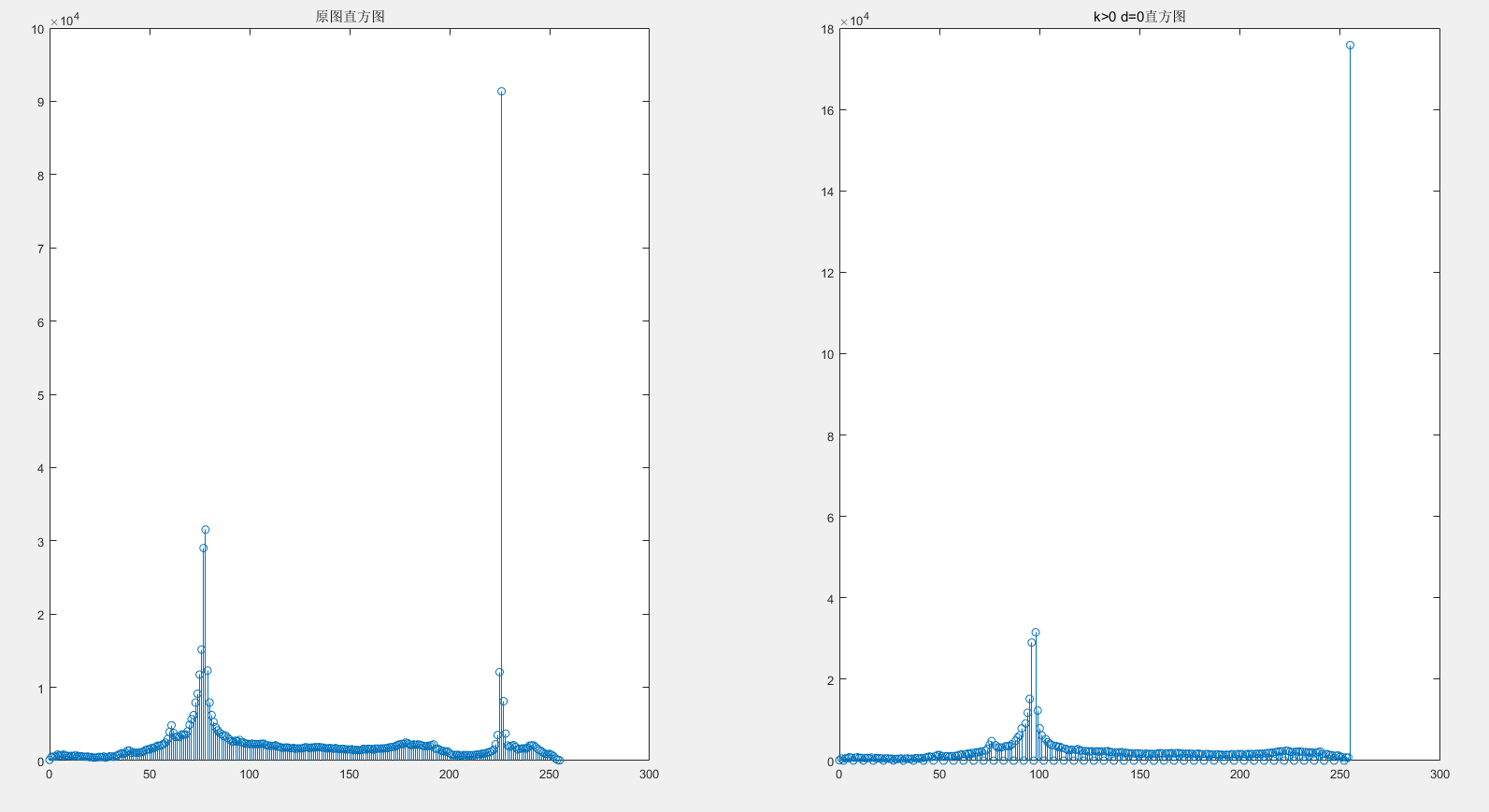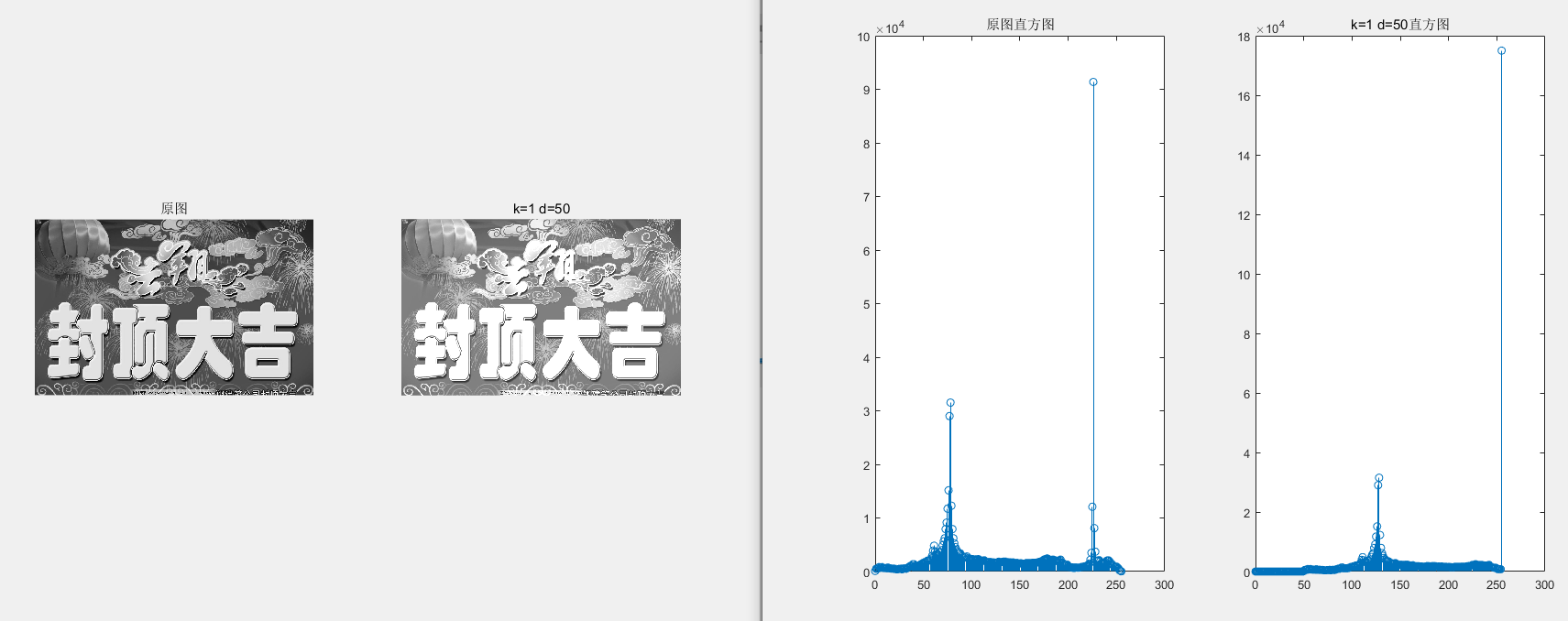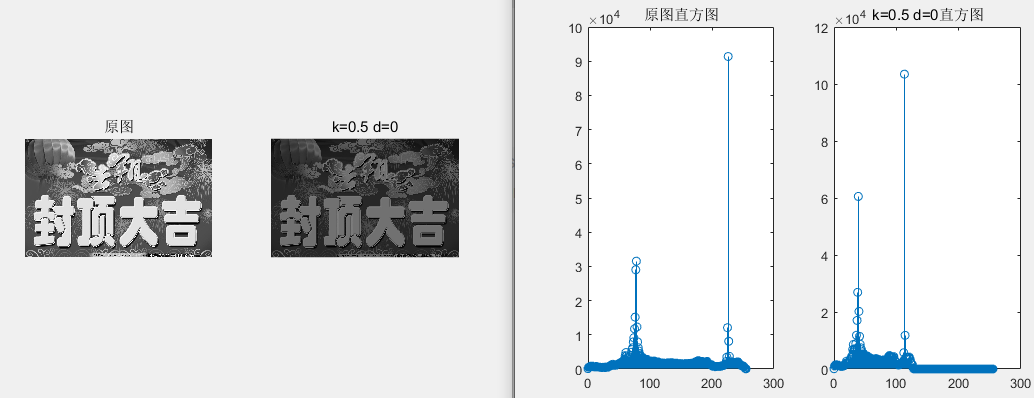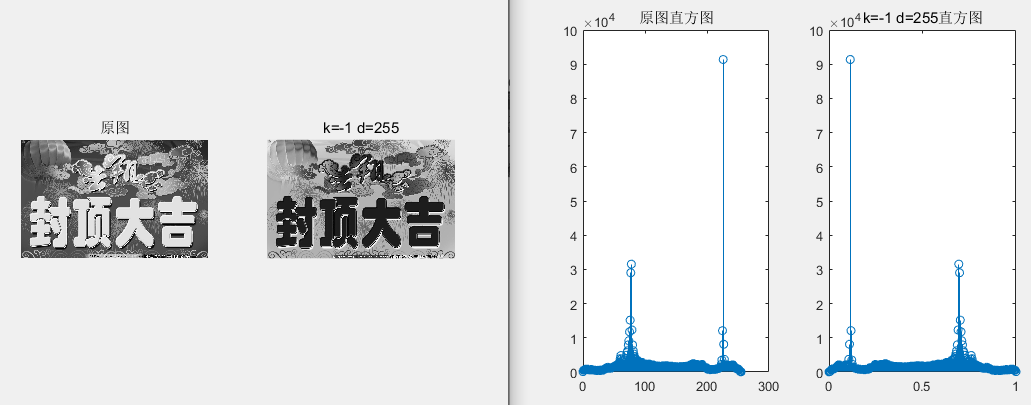# 7 讨论

2015-04-12 10:45:08 Trent1985 阅读数 1305
• ###### 数据结构基础系列(2)：线性表

数据结构课程是计算机类专业的专业基础课程，在IT人才培养中，起着重要的作用。课程按照大学计算机类专业课程大纲的要求，安排教学内容，满足需要系统学习数据结构的人。系列课程包含11个部分，本课为第2部分，线性表的逻辑结构，线性表的顺序表和链表两种存储结构，以及在各种存储结构中基本运算的实现，还通过相关的应用案例介绍了相关知识的应用方法。

19186 人正在学习 去看看 贺利坚
﻿﻿

[函数名称][函数代码]
/// <summary>
/// Nonlinear transform process.
/// </summary>
/// <param name="src">The source image.</param>
/// <param name="k">Param to adjust nonlinear transform, from 0 to 255.</param>
/// <returns></returns>
public static WriteableBitmap NonlinearTransformProcess(WriteableBitmap src,int k )////37
{
if (src != null)
{
int w = src.PixelWidth;
int h = src.PixelHeight;
WriteableBitmap linearImage = new WriteableBitmap(w, h);
byte[] temp = src.PixelBuffer.ToArray();
int r = 0, g = 0, b = 0;
for (int i = 0; i < temp.Length; i += 4)
{
b = (int)(k * Math.Log10(1 + temp[i]));
g = (int)(k * Math.Log10(1 + temp[i + 1]));
r = (int)(k * Math.Log10(1 + temp[i + 2]));
temp[i] = (byte)(b > 0 ? (b < 255 ? b : 255) : 0);
temp[i + 1] = (byte)(g > 0 ? (g < 255 ? g : 255) : 0);
temp[i + 2] = (byte)(r > 0 ? (r < 255 ? r : 255) : 0);
}
Stream sTemp = linearImage.PixelBuffer.AsStream();
sTemp.Seek(0, SeekOrigin.Begin);
sTemp.Write(temp, 0, w * 4 * h);
return linearImage;
}
else
{
return null;
}
}2014-12-10 22:57:57 whustyle 阅读数 1564
• ###### 数据结构基础系列(2)：线性表

数据结构课程是计算机类专业的专业基础课程，在IT人才培养中，起着重要的作用。课程按照大学计算机类专业课程大纲的要求，安排教学内容，满足需要系统学习数据结构的人。系列课程包含11个部分，本课为第2部分，线性表的逻辑结构，线性表的顺序表和链表两种存储结构，以及在各种存储结构中基本运算的实现，还通过相关的应用案例介绍了相关知识的应用方法。

19186 人正在学习 去看看 贺利坚

承接上一篇博客，接下来实现以下灰度图像的线性拉伸变换，具体理论就不赘述了，学过数字图像处理的基本都知道，下面来看看如何通过VC6.0的MFC编程实现它。void CImageProcessView::OnLinertrans()
{

}

下面，我们需要创建一个对话框，来接受用户设置的线性变换参数，最小值和最大值，可以右键Dialog，InsertDialog，设置对话框ID为：IDD_DIALOG_LinerTransSet为对话框创建类，双击对话框，提示创建新的类，选择确定，设置类参数如下：这些准备工作做好了之后，便可以实现前面空着的OnLinertrans函数了，先在View.cpp文件上方添加头文件包含# include "LinerTransDlg.h"，然后实现OnLinertrans，

void CImageProcessView::OnLinertrans()
{
CLinerTransDlg linedlg;

if(linedlg.DoModal()==IDOK)
{
UpdateData(TRUE);
CImageProcessDoc* pDoc=GetDocument();
ASSERT_VALID(pDoc);

BYTE* pData=pDoc->m_bmpFile.m_pImgDat;

int cols=pDoc->m_bmpFile.m_Cols;
int rows=pDoc->m_bmpFile.m_Rows;
int min=linedlg.m_nMin;
int max=linedlg.m_nMax;

float mingray=255,maxgray=0;

for(int r=0;r<rows;r++)
{
for(int c=0;c<cols;c++)
{
if(*(pData+cols*r+c)<mingray)
mingray=*(pData+cols*r+c);

if(*(pData+cols*r+c)>maxgray)
maxgray=*(pData+cols*r+c);

}
}
float a=(min-max)/(mingray-maxgray);
for(r=0;r<rows;r++)
{
for(int c=0;c<cols;c++)
{
int gray=int(min+a*(*(pData+cols*r+c)-mingray));
*(pData+cols*r+c)=BYTE(gray);
}
}
Invalidate();
}
}2019-03-31 15:31:25 Eastmount 阅读数 16963
• ###### 数据结构基础系列(2)：线性表

数据结构课程是计算机类专业的专业基础课程，在IT人才培养中，起着重要的作用。课程按照大学计算机类专业课程大纲的要求，安排教学内容，满足需要系统学习数据结构的人。系列课程包含11个部分，本课为第2部分，线性表的逻辑结构，线性表的顺序表和链表两种存储结构，以及在各种存储结构中基本运算的实现，还通过相关的应用案例介绍了相关知识的应用方法。

19186 人正在学习 去看看 贺利坚

PS：请求帮忙点个Star，哈哈，第一次使用Github，以后会分享更多代码，一起加油。

1.图像灰度非线性变换：DB=DA×DA/255
2.图像灰度对数变换
3.图像灰度伽玛变换

PS：文章参考自己以前系列图像处理文章及OpenCV库函数，同时参考如下文献：

《数字图像处理》（第3版），冈萨雷斯著，阮秋琦译，电子工业出版社，2013年.
《数字图像处理学》（第3版），阮秋琦，电子工业出版社，2008年，北京.
《OpenCV3编程入门》，毛星云，冷雪飞，电子工业出版社，2015.
[数字图像处理] 五.MFC图像点运算之灰度线性变化、灰度非线性变化、阈值化和均衡化处理详解
python+opencv+图像特效（图像灰度处理、颜色翻转、图片融合，边缘检测，浮雕效果）

OpenCV图像增强算法实现（直方图均衡化、拉普拉斯、Log、Gamma）

# 一.图像灰度非线性变换：DB=DA×DA/255

# -*- coding: utf-8 -*-
import cv2
import numpy as np
import matplotlib.pyplot as plt

#读取原始图像

#图像灰度转换
grayImage = cv2.cvtColor(img, cv2.COLOR_BGR2GRAY)

#获取图像高度和宽度
height = grayImage.shape
width = grayImage.shape

#创建一幅图像
result = np.zeros((height, width), np.uint8)

#图像灰度非线性变换：DB=DA×DA/255
for i in range(height):
for j in range(width):
gray = int(grayImage[i,j])*int(grayImage[i,j]) / 255
result[i,j] = np.uint8(gray)

#显示图像
cv2.imshow("Gray Image", grayImage)
cv2.imshow("Result", result)

#等待显示
cv2.waitKey(0)
cv2.destroyAllWindows()# 二.图像灰度对数变换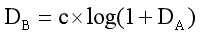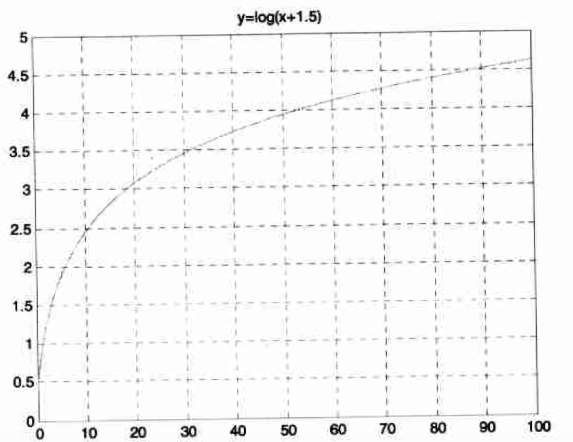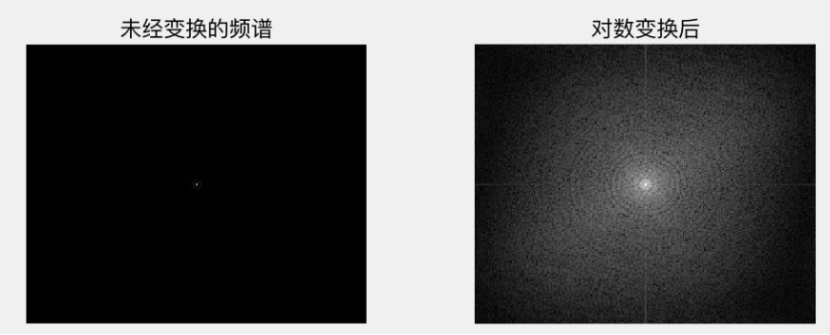# -*- coding: utf-8 -*-
import numpy as np
import matplotlib.pyplot as plt
import cv2

#绘制曲线
def log_plot(c):
x = np.arange(0, 256, 0.01)
y = c * np.log(1 + x)
plt.plot(x, y, 'r', linewidth=1)
plt.rcParams['font.sans-serif']=['SimHei'] #正常显示中文标签
plt.title(u'对数变换函数')
plt.xlim(0, 255), plt.ylim(0, 255)
plt.show()

#对数变换
def log(c, img):
output = c * np.log(1.0 + img)
output = np.uint8(output + 0.5)
return output

#读取原始图像

#绘制对数变换曲线
log_plot(42)

#图像灰度对数变换
output = log(42, img)

#显示图像
cv2.imshow('Input', img)
cv2.imshow('Output', output)
cv2.waitKey(0)
cv2.destroyAllWindows()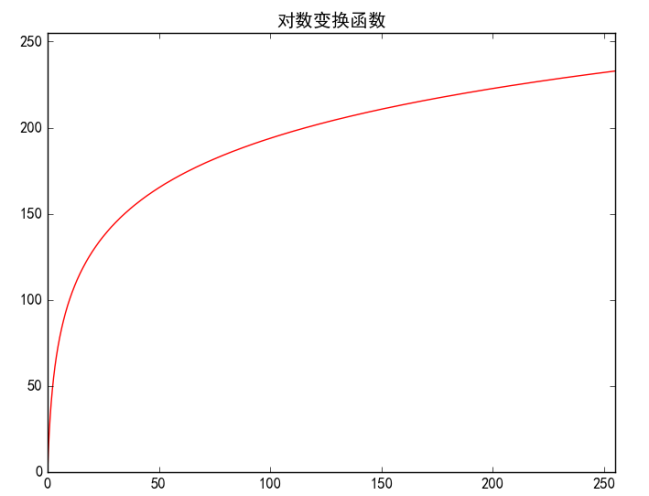# 三.图像灰度伽玛变换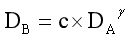• 当γ>1时，会拉伸图像中灰度级较高的区域，压缩灰度级较低的部分。
• 当γ<1时，会拉伸图像中灰度级较低的区域，压缩灰度级较高的部分。
• 当γ=1时，该灰度变换是线性的，此时通过线性方式改变原图像。

Python实现图像灰度的伽玛变换代码如下，主要调用幂函数实现。

# -*- coding: utf-8 -*-
import numpy as np
import matplotlib.pyplot as plt
import cv2

#绘制曲线
def gamma_plot(c, v):
x = np.arange(0, 256, 0.01)
y = c*x**v
plt.plot(x, y, 'r', linewidth=1)
plt.rcParams['font.sans-serif']=['SimHei'] #正常显示中文标签
plt.title(u'伽马变换函数')
plt.xlim([0, 255]), plt.ylim([0, 255])
plt.show()

#伽玛变换
def gamma(img, c, v):
lut = np.zeros(256, dtype=np.float32)
for i in range(256):
lut[i] = c * i ** v
output_img = cv2.LUT(img, lut) #像素灰度值的映射
output_img = np.uint8(output_img+0.5)
return output_img

#读取原始图像

#绘制伽玛变换曲线
gamma_plot(0.00000005, 4.0)

#图像灰度伽玛变换
output = gamma(img, 0.00000005, 4.0)

#显示图像
cv2.imshow('Imput', img)
cv2.imshow('Output', output)
cv2.waitKey(0)
cv2.destroyAllWindows()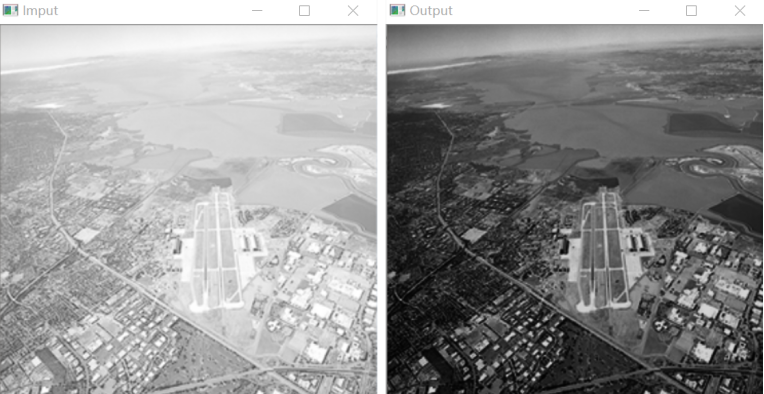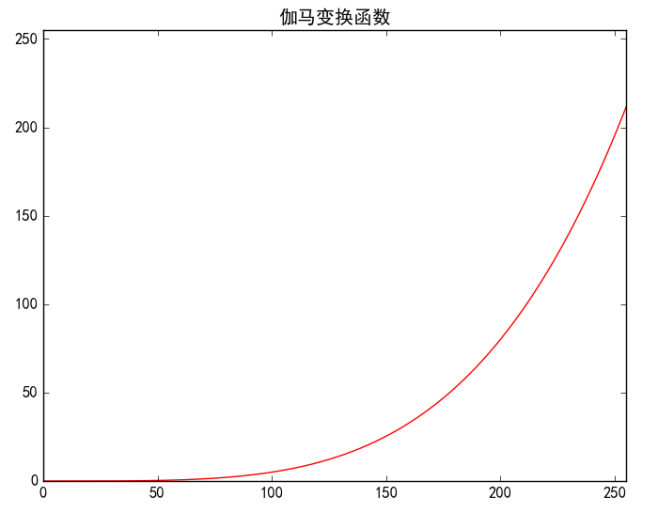（By：Eastmount 2019-03-31 深夜12点 https://blog.csdn.net/Eastmount/）

2018-03-13 10:00:00 weixin_33981932 阅读数 15
• ###### 数据结构基础系列(2)：线性表

数据结构课程是计算机类专业的专业基础课程，在IT人才培养中，起着重要的作用。课程按照大学计算机类专业课程大纲的要求，安排教学内容，满足需要系统学习数据结构的人。系列课程包含11个部分，本课为第2部分，线性表的逻辑结构，线性表的顺序表和链表两种存储结构，以及在各种存储结构中基本运算的实现，还通过相关的应用案例介绍了相关知识的应用方法。

19186 人正在学习 去看看 贺利坚

﻿﻿

2.8图像线性变换

[函数名称][函数代码]

///<summary>

/// Linear transform process(f=kf+v).

///</summary>

///<param name="src">Source image.</param>

///<param name="k">Parameter,from 0 to 5.</param>

///<param name="v">Parameter,from -128 to 128.</param>

///<returns></returns>

publicstaticWriteableBitmap LinearTransformProcess(WriteableBitmap src,double k,int v)////8线性变换处理

{

if(src!=null )

{

int w = src.PixelWidth;

int h = src.PixelHeight;

WriteableBitmap linearImage =newWriteableBitmap(w,h);

byte[] temp = src.PixelBuffer.ToArray();

for (int i = 0; i < temp.Length; i +=4)

{

temp[i] = (byte)(((k * temp[i] + v + 0.5) > 255 ? 255 : (k * temp[i] + v + 0.5)) < 0 ? 0 : ((k * temp[i] + v + 0.5) > 255 ? 255 : (k * temp[i] + v + 0.5)));

temp[i+1] = (byte)(((k * temp[i+1] + v + 0.5) > 255 ? 255 : (k * temp[i+1] + v + 0.5)) < 0 ? 0 : ((k * temp[i+1] + v + 0.5) > 255 ? 255 : (k * temp[i+1] + v + 0.5)));

temp[i+2] = (byte)(((k * temp[i+2] + v + 0.5) > 255 ? 255 : (k * temp[i+2] + v + 0.5)) < 0 ? 0 : ((k * temp[i+2] + v + 0.5) > 255 ? 255 : (k * temp[i+2] + v + 0.5)));

}

Stream sTemp = linearImage.PixelBuffer.AsStream();

sTemp.Seek(0,SeekOrigin.Begin);

sTemp.Write(temp, 0, w * 4 * h);

return linearImage;

}

else

{

returnnull;

}

}

[图像效果]